## Exploring paths for Sudoku variants with Python

Published January 9, 2021 on Chandler Swift's Blog Source

One of my college friends has an interest in Sudoku variants. Generally, that manifests in the two of us solving puzzles curated by Cracking the Cryptic, but occasionally it ends up with me trying to solve a puzzle he’s created. A while back, he and I were chatting about one of his ideas for the basis of a puzzle. It’s based around a snake in the grid (for an example of another puzzle using a snake, check out this puzzle by CtC). His puzzle requires that its snake alternates successive even digits and odd digits, is unambiguous, and visits at least one cell in every box (as well as, of course, being a valid sudoku puzzle!).

Let’s talk about those rules in a bit more depth:

• Snake: A snake is a one-wide orthogonally connected path. Much like in the classic Snake game, it can’t touch itself anywhere along its length (although diagonal adjacency is fine). The one in this puzzle has alternating even and odd digits along its length, represented in this post by red and blue respectively: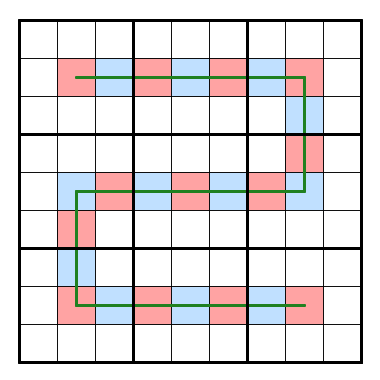• visits a cell in every box: The above snake fills this requirement as well. The following (shorter) snake does, too: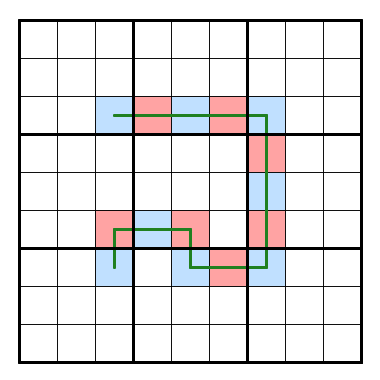• and the big constraint, is unambiguous: Given the pattern of even and odd digits in the grid, there is exactly one direction the snake could continue at any point and still satisfy the parity constraint. To see this happen, we also have to have some information about the parity of the cells surrounding the snake. In general, each cell of the snake is touching either one (if it’s the head/tail) or two (if it’s part of the body) other cells that are snake cells. These must be of the opposite parity. The other cells must be of the same parity.

For example, suppose that the head of the snake is even, and continues into the (odd) cell to its right: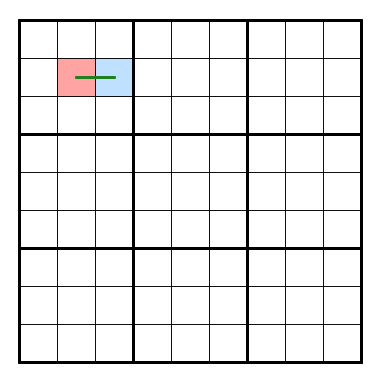To ensure there’s no ambiguity that the snake continues to the right, all of the other cells must be invalid for the snake to enter; that is, they must all have the same parity as the head: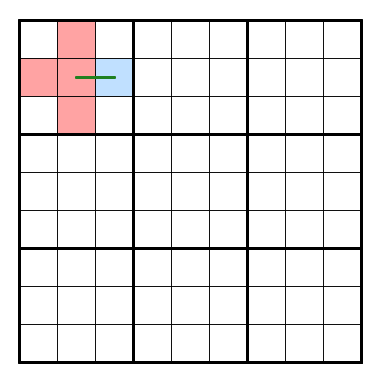Now, each box must contain a 1 through 9; that is, four even and five odd digits. This one already contains all four even digits, so the unfilled cells must be odd: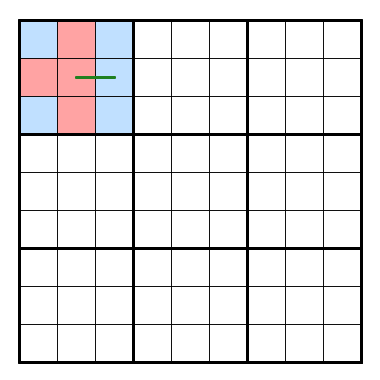The only place the snake can proceed, then, is directly into the second box: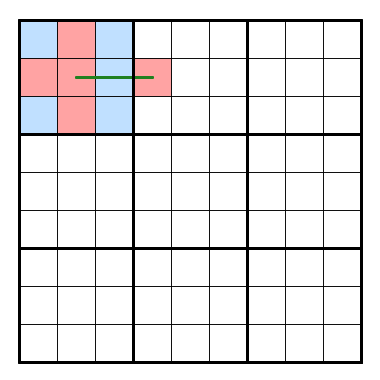If we continue to the right, we would need at least five red cells in the top middle box (try it out, if you like!) so now we have to move down…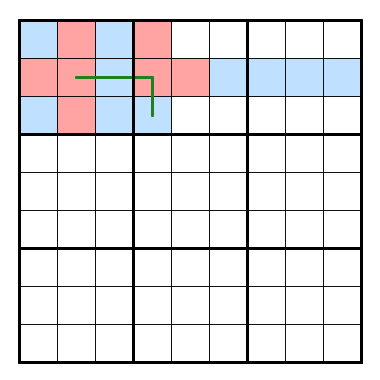Repeating this logic eventually gets us here. This scenario, however, is already broken—there simply isn’t a way to get the snake back into the top right box, if we can’t put a red cell in that middle row.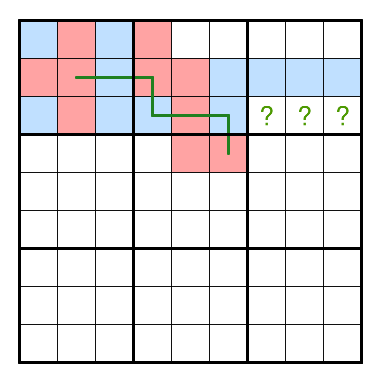This type of logic is fairly time-consuming and error prone, but it’s not really that complex1, so we put together a Python program to try out paths for us that satisfy the even-odd and uniqueness constraints. Given that it was written hastily to solve a one-time problem, it’s certainly neither very neat nor readable, but it seems to do what we want!

Open if you dare!
``````#!/usr/bin/python3
import copy
from time import sleep

# Originally these were just letters (X and O for even and odd snake, and x and
# o for even and odd empty cell), but as long as they're distinct, they do what
# we need. So these include ANSI color codes, which colors the snake red and
# blue.
EMPTY = "_"
ODD = "\033[34m_\033[0m"
EVEN = "\033[31m_\033[0m"
ODD_SNAKE = "\033[34mX\033[0m"
EVEN_SNAKE = "\033[31mX\033[0m"

# Here we define a few helper functions to make our lives easier later.
attempts = 0
def print_grid(grid):
global attempts
print(f"Attempt {attempts}:")
attempts += 1
for row in range(9):
print(" ".join([r[row] for r in grid]))
print()

def opposite_parity(x):
if x == EVEN:
return ODD
else:
return EVEN

def snake(x):
if x == EVEN:
return EVEN_SNAKE
else:
return ODD_SNAKE

"""
cells are filled with snake. If one is filled, we must be at the head of a
snake; if two are filled we're along the body. No more than two can be
filled, because then we'd have broken the snake; it would fork.
"""
if cell > 0 and grid[cell][cell - 1] in [ODD_SNAKE, EVEN_SNAKE]: # north

if cell < 8 and grid[cell + 1][cell] in [ODD_SNAKE, EVEN_SNAKE]: # east

if cell < 8 and grid[cell][cell + 1] in [ODD_SNAKE, EVEN_SNAKE]: # south

if cell > 0 and grid[cell - 1][cell] in [ODD_SNAKE, EVEN_SNAKE]: # west

def check_grid_parity(grid):
"""
check_grid_parity employs a multi-pass approach. For each pass, it counts
the even and odd cells in each row, column, and box. Then, if it has
either four even or five odd cells, it knows that all of the others must be
of the opposite parity, so it fills them in. This, however, may complete
other rows/columns/boxes, so we'll do another pass after this one is done.
We continue to do passes until either something breaks (returning False),
or nothing is left to change.

This is a lot of repetitive but just-slightly-different-each-time logic, so
it can't easily be condensed. However, it's not particularly complicated,
just rather verbose.
"""
do_another_pass=False
# check each row
for row in grid:
even_count = odd_count = 0
for cell in row:
if cell == EVEN or cell == EVEN_SNAKE:
even_count += 1
elif cell == ODD or cell == ODD_SNAKE:
odd_count += 1
if even_count > 4 or odd_count > 5:
#print("broken on row")
return False
elif even_count == 4:
for cell_index in range(len(row)):
if row[cell_index] == EMPTY:
#print("filling row with odd")
row[cell_index] = ODD
do_another_pass=True
elif odd_count == 5:
for cell_index in range(len(row)):
if row[cell_index] == EMPTY:
#print("filling row with even")
row[cell_index] = EVEN
do_another_pass=True

# check each column
for col_index in range(9):
even_count = odd_count = 0
for row in grid:
if row[col_index] == EVEN or row[col_index] == EVEN_SNAKE:
even_count += 1
elif row[col_index] == ODD or row[col_index] == ODD_SNAKE:
odd_count += 1
#print(f"row {col_index}: even: {even_count}, odd: {odd_count}")
if even_count > 4 or odd_count > 5:
#print("broken on column")
return False
elif even_count == 4:
for row in grid:
if row[col_index] == EMPTY:
row[col_index] = ODD
#print("filling col with odd")
do_another_pass=True
elif odd_count == 5:
for row in grid:
if row[col_index] == EMPTY:
row[col_index] = EVEN
#print("filling col with even")
do_another_pass=True

# check each box
for box_x in range(3):
for box_y in range(3):
even_count = odd_count = 0
for x in range(3*box_x, 3*box_x+3):
for y in range(3*box_y, 3*box_y + 3):
if grid[x][y] == EVEN or grid[x][y] == EVEN_SNAKE:
even_count += 1
elif grid[x][y] == ODD or grid[x][y] == ODD_SNAKE:
odd_count += 1
if even_count > 4 or odd_count > 5:
return False
elif even_count == 4:
for x in range(3*box_x, 3*box_x+3):
for y in range(3*box_y, 3*box_y + 3):
if grid[x][y] == EMPTY:
grid[x][y] = ODD
#print("filling box with odd")
do_another_pass=True
elif odd_count == 5:
for x in range(3*box_x, 3*box_x+3):
for y in range(3*box_y, 3*box_y + 3):
if grid[x][y] == EMPTY:
grid[x][y] = EVEN
#print("filling box with even")
do_another_pass=True

if do_another_pass:
return check_grid_parity(grid)
else:
return True

if cell > 0 and grid[cell][cell - 1] == parity: # north

if cell < 8 and grid[cell + 1][cell] == parity: # east

if cell < 8 and grid[cell][cell + 1] == parity: # south

if cell > 0 and grid[cell - 1][cell] == parity: # west

def fill_empty_cells_around(grid, cell, value):
"""
fill_empty_cells_around is applied to the "neck" (that is, the cell next to
the head) of the snake. Supposing we have a snake like this, where E and O
represent even and odd snake cells, and e and o non-snake cells:
e o e _
E O E O E
e o e _
then fill_empty_cells_around will be used with the above as `grid`, the
last O in the row as `cell`, and "o" as `value`.
"""

if cell > 0 and grid[cell][cell - 1] == opposite_parity(value): # north
raise ValueError
elif cell > 0 and grid[cell][cell - 1] == EMPTY: # north
grid[cell][cell - 1] = value

if cell < 8 and grid[cell + 1][cell] == opposite_parity(value): # east
raise ValueError
elif cell < 8 and grid[cell + 1][cell] == EMPTY: # east
grid[cell + 1][cell] = value

if cell < 8 and grid[cell][cell + 1] == opposite_parity(value): # south
raise ValueError
elif cell < 8 and grid[cell][cell + 1] == EMPTY: # south
grid[cell][cell + 1] = value

if cell > 0 and grid[cell - 1][cell] == opposite_parity(value): # west
raise ValueError
elif cell > 0 and grid[cell - 1][cell] == EMPTY: # west
grid[cell - 1][cell] = value

# disable this if you don't want to see the solving visualization. Then you
# can also disable the sleep(10) about 20 lines below, as we'll only be
# printing the solutions.
print_grid(grid)

# check if we broke the grid:
if not check_grid_parity(grid):
return # try the next case

# give the terminal a chance to keep up. On a 60hz display, this should
# leave the grid on display for 1-2 frames.
sleep(0.03)

# base case: have we solved it (reached every box at least once)
snakes_everywhere = True
for box_x in range(3):
for box_y in range(3):
snake_in_grid = False
for x in range(3*box_x, 3*box_x+3):
for y in range(3*box_y, 3*box_y + 3):
if grid[x][y] in [ODD_SNAKE, EVEN_SNAKE]:
snake_in_grid = True
if not snake_in_grid:
snakes_everywhere = False
if (snakes_everywhere and
print("Solved!")
print_grid(grid)
sleep(10) # give us a chance to look at what we found
# note that we don't return here; we can try to see if there's a longer
# snake that's also a solution.

# if the cell is empty, then it doesn't have a parity, and therefore a snake
# does not pass by in an adjacent cell, so it's a valid cell to try to enter
#
# For each of the directions (ordered north, east, south, and west), we make
# sure we're not along that wall (if we're along the north wall, of course
# we can't go north); we verify that either cell to the \$direction of the
# current head is empty (no constraints, so we can enter), or that it's the
# same color as the one we're entering _and_ doesn't have two adjacent
# snakes (that is, another besides the one we're entering from).
#
# If we have a neck cell (which we always do except on the very first cell
# of the snake), we fill the cells around the neck with the appropriate
# color. Note that we're filling the cells around the neck and not the
# head. Although it does take one step longer to fail than would otherwise
# be necessary, this saves us a lot of headache later: Say our head is even.
# Then all of the cells around it except the neck cell (if applicable) and
# the cell that will be the head next iteration (if the snake is not
# complete) will also be even, to make the snake unambiguous. However, then
# next iteration we will need to continue the snake into one of those even
# cells---which one? Conversely, if we simply apply it to the neck after the
# location of the new head has been decided, we avoid all this difficulty.
#
# We repeat this process for each possible direction.
new_grid = copy.deepcopy(grid)
if neck is not None:
try:
fill_empty_cells_around(new_grid, neck, opposite_parity(last_parity))
except ValueError:  # Cell is required to be both even and odd---abort!
return # the neck is broken so this _can't_ be the head

new_grid = copy.deepcopy(grid)
if neck is not None:
try:
fill_empty_cells_around(new_grid, neck, opposite_parity(last_parity))
except ValueError:  # Cell is required to be both even and odd---abort!
return

new_grid = copy.deepcopy(grid)
if neck is not None:
try:
fill_empty_cells_around(new_grid, neck, opposite_parity(last_parity))
except ValueError:  # Cell is required to be both even and odd---abort!
return

new_grid = copy.deepcopy(grid)
if neck is not None:
try:
fill_empty_cells_around(new_grid, neck, opposite_parity(last_parity))
except ValueError:  # Cell is required to be both even and odd---abort!
return

# We attempt to start in each cell in the grid, though to cut our search space
# and time in half, we constrain start_y <= start_x. Any snakes that would be
# found with start_y > start_x will be a mirror of one that will be found here.
# This still leaves quite a few duplicates by reflection and rotation, but those
# will be harder to weed out by simply adjusting the ranges here.
for start_x in range(9):
for start_y in range(start_x + 1):
empty_grid = []
for i in range(9):
empty_grid.append([EMPTY] * 9)
empty_grid[start_x][start_y] = ODD_SNAKE
recursive_dfs(empty_grid, (start_x, start_y), None, ODD)
``````

While the rate my terminal emulator can display the text seems to currently be the bottleneck, it’s still certainly not slow, so I left the output displayed at each step the algorithm takes. This was essential as a debugging tool, but now it’s just nice to watch it run. It’s really a rather pretty visual; I forgot how much time I could spend staring at visualizations of searches2! If I’m ever bored for an evening, I may consider porting it to Javascript so it can run in the browser, but for now if you want to see more, you’ll just have to download and run it yourself. All you need is Python.

A version of this program with all the print statements removed took about five minutes to find 3241 possible configurations that satisfied the constraints.

Since we’ve found all the possible combinations (recognized by this program, at least), we can also figure out the shortest and longest possible paths that satisfy the constraints—21 and 37, respectively. And both are pretty neat shapes!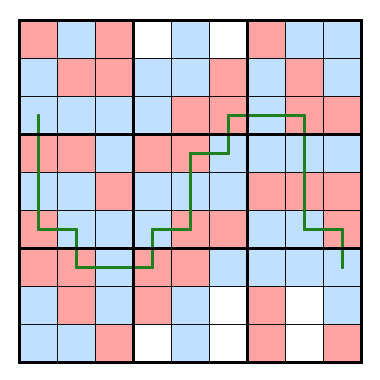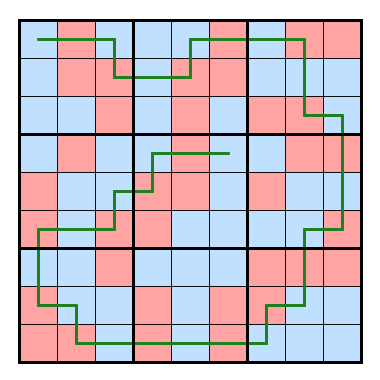Images from this post were created using penpa-edit.

1. Over-optimistic as always, I think I had said we’d probably have a solution together in an hour. I think by the time we’d worked out all the bugs and edge cases, we were somewhere between three and four hours in, but it was a fun challenge to write! ↩︎

2. If you also enjoy watching this sort of thing, Jamis Buck’s page on mazes has a bunch of really neat animations! ↩︎

I don't have a formal commenting system set up. If you have questions or comments about anything I've written, send me an email and I'd be delighted to hear what you have to say!